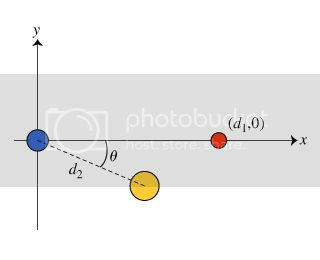# [SOLVED] change from rectangular to cylindrical coordinates. (let r ≥ 0 and 0 ≤ θ ≤ 2π.)

edited

There are three spheres: red, yellow, and blue. Blue is on the origin, red at d1,0, and yellow at (d2cos(theta),-d2sin(theta))

Suppose that the magnitude of the charge on the yellow sphere is determined to be 2q. Calculate the charge Qred on the red sphere.

I have the two force components necessary to solve the equation figured out. Once again, my trouble is putting them together to solve for charge Q, something that has not been covered in my course yet.

Here are my two force equations:

Fx(yellow)=k*2*q^2*cos(theta)/(d_2)^2

Fx(red)=-k*q_red*q/d_1^2

q_red=... is what I am trying to find.

Update:This is a link to a picture that might help

Update 2:

The charge on blue is positive.

The charge on yellow is Negative

Charge on red is positive

Update 3:

Consider the following configuration of fixed, uniformly charged spheres:

* a blue sphere fixed at the origin with positive charge q.

* a red sphere fixed at the point (d_1,,0) with unknown charge q_red,

* a yellow sphere fixed at the point (d_2cos(theta),,-d_2sin(theta)) with unknown charge q_yellow.

The net electric force on the blue sphere is observed to be vec{F} = (0, \- F), where F > 0

Update 4:

My answer for q_red has to be in terms of

q,d1,d2, and theta

Not some constant but instead variables.

Update 5:

solved by ukmudgal

by
selected by

A blue sphere is fixed at the origin with positive charge q; a red sphere fixed at the point (d1,0) with unknown charge qred; yellow sphere fixed at the point (d2,cos (theta),-d2 sin (theta))with unknown charge qyellow.

The x component of position vector of yellow sphere is positive (d2cos (theta)).

The y component of position vector of yellow sphere is negative ( -d2sin (theta)).

The net electric force on the blue sphere is observed to be F(vector)=(0,-F), where F>0.

As q'blue' and q'red' are on x - axis and the force between them will have no component along y - axis

The net force on charged blue sphere is along negative y - axis and the y component of position vector of yellow sphere is negative ( -d2sin (theta)).

Hence the yellow sphere must be attracting blue sphere,which means,nature of charge on yellow sphere is opposite to nature of charge on blue sphere.

Charge on blue sphere is positive,hence charge on yellow sphere is negative

A) The sign of the charge on the yellow sphere is negative

______________________________________...

B) As the component of net force on x - axis is zero,the x component of force on blue due to yellow must be equal and opposite to force on blue due to red

In other words, as yellow attracts blue and red must repel blue.

Hence nature of charge on q'red' is opposite to nature of charge on q'yellow'

Charge on yellow sphere is negative ,hence charge on red is positive

B) The sign of the charge on the red sphere is

positive

______________________

Edited

As x component of resultant force is zero,

Fx,yellow cos theta = Fx,red

[ k(2q)q/(d2)^2] cos theta =kqred q /(d1)^2

(2q)/(d2)^2] cos theta =qred /(d1)^2

[(2q) (d1)^2 /(d2)^2] cos theta =qred

q red = (2q)* cos theta* [d1 /d2]^2

the charge Qred on the red sphere is (2q)* cos theta* [d1 /d2]^2

Lorem ipsum dolor sit amet, consectetur adipiscing elit, sed do eiusmod tempor incididunt ut labore et dolore magna aliqua. Ut enim ad minim veniam, quis nostrud exercitation ullamco laboris nisi ut aliquip ex ea commodo consequat. Duis aute irure dolor in reprehenderit in voluptate velit esse cillum dolore eu fugiat.

+1 vote